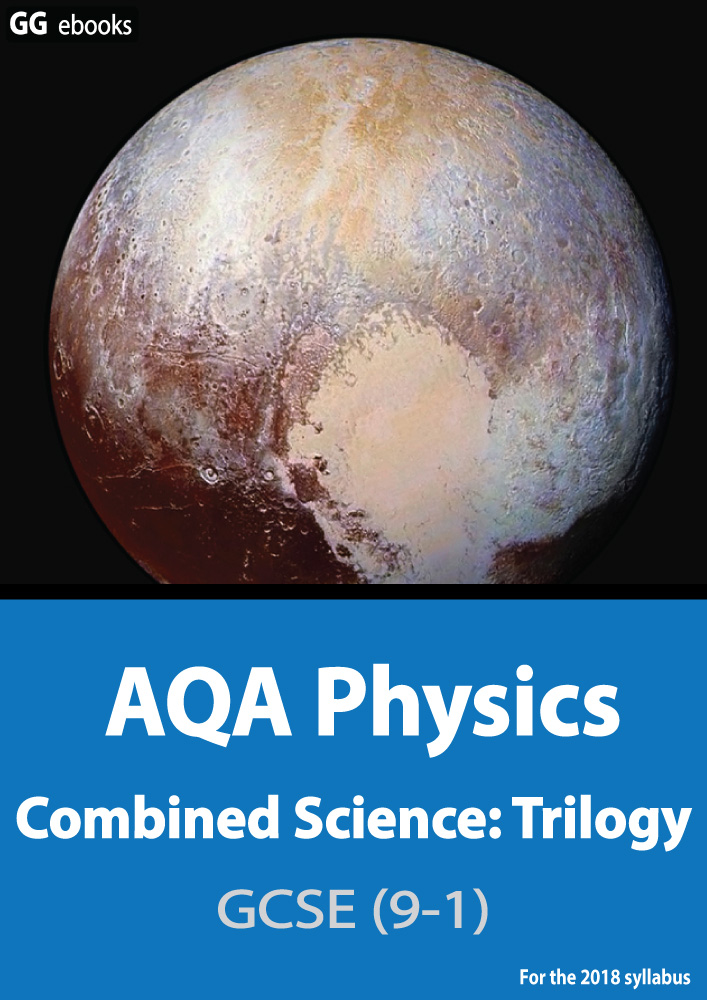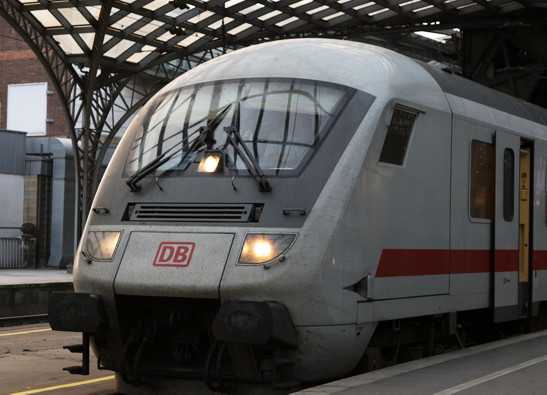NEED HELP? AQA GCSE digital textbooks From the makers of Grade Gorilla GGebooks.com(Opens in a new tab)1. Work done is measured in

• A) joules
• B) watts
• C) coulombs
• D) newtons

2. Which of these is NOT a store of energy?

• A) chemical
• B) gravitational potential
• C) friction
• D) kinetic

3-9. Choose the correct energy store in the following systems:

 A bow and arrow transfers ? thermal kinetic gravitational nuclear chemical elastic energy into useful ? thermal kinetic gravitational nuclear chemical elastic energy. A petrol engine transfers ? thermal kinetic gravitational nuclear chemical elastic energy into useful ? thermal kinetic gravitational nuclear chemical elastic energy. Most of the energy wasted is lost as ? thermal kinetic gravitational nuclear chemical elastic energy. A falling ball transfers ? thermal kinetic gravitational nuclear chemical elastic energy into ? thermal kinetic gravitational nuclear chemical elastic energy.

A motor on a train converts 60 000J of electrical energy into 45 000J of kinetic energy as the train accelerates.10. What is 60 000J in kJ?
11. How efficient is the motor?
12. If it converts this energy in 15 seconds, what is the useful power output?

13. What is the formula for work done?

• A) Work done = force x distance
• B) Work done = force ÷ distance
• C) Work done = mass x acceleration
• D) Work done = mass ÷ acceleration

14. A tractor pulls a plough 2km across a field using a force of 8kN. What is the work done?

15-16. What are the missing labels on this energy flow diagram for a radio?

 Electrical Energy 5000J? Potential energy Kinetic energy Sound energy Light energy 800J
 Thermal energy ? 4200J 4920J 5000J 5800J